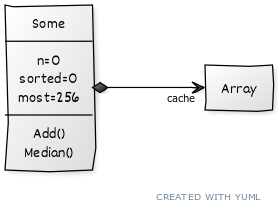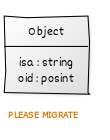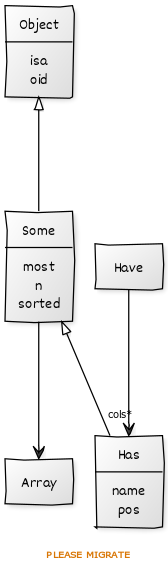# Objects

This page introduces GOLD’s object system, via example. In the following, note that:

• Class names are lower case with one upper case, leading letter;
• e.g. “`Some`” (see below).
• Object constructors are functions with the same name as the class;
• e.g. see the “`Some`” function (below).
• Object methods are functions with two upper case letters. Each method has to start with a class name;
• e.g. see the “`SomeAdd`” function (below).
• Object attribute accessors are implemented with a dot notation. These accessors can be nested;
• e.g. “`a.b.c.d`”.

The `Some` class described below generates objects that store up to `most` items, in an associated `cache` array.Note that GOLD constructors’ first act is a call to a super class constructor (and the top-level built-in constructor is `Object`).

``````function Some(i,     most) {
isa(Object(i))
i.most = either(most,256) # keep up to `most` number of items
i.n = 0
i.sorted = 0
has(i,"cache")            # i.cache holds the kept value
}
``````

Whenever we add a new items, the `sorted` flag gets set to false (which, GAWK-speak, means `sorted=0`). If our cache is full, then we replace items at random. This function returns (0 or 1) according to whether or the function (ignores or keeps) the added item, respectively.

``````function SomeAdd(i,v,    added,len) {
i.n++
i.sorted = 0
len = length(i.cache)
if (len < i.most) {  # the cache is not full, add something
push(i.cache,v)
} else if (rand() < len/i.n) {  # else, sometimes, add "v"
i.cache[ int(len*rand()) + 1 ] = v
}
}
``````

If ever we want the median of the numbers, first we check if everything is sorted. If not, we sort the `cache` then call a standard `median` function on that `cache`.

``````function SomeMedian(i,  m,n) {
n= i.sorted ? length(i.cache) : asort(i.cache)
i.sorted=1
return median(i.cache)
}
``````

## Inside GOLD

All the following code comes with the file `gold.gold` (created by the GOLD installation procedure).

### The `Object` Base Class

GOLD’s built-in top-level class is `Object`. In the `Object` constructor:

• The `new` function (shown below), creates a new empty array.
• Then GOLD adds a unique identifier called `oid` for each object;
• And a text flag describing the class (`isa`).``````function Object(i) {
new(i)
i["isa"] = "Object"
i["oid"] = ++OID
}

function new(i) { split("",i,"") }
``````

### Aggregation (one-to-one)

In the above `Some` constructor, the `has` function adds a nested array in the `cache` field. The trick to adding nested arrays in GAWK is:

• The first time that field is references, then make it an array. Then use `split` to make sure that array is an empty array.
• Optionally, pass down a constructor to fill in that space. If no such constructor is passed, then we just leave the field is an array.
``````# constructor takes zero arguments
function has(lst,key,fun) {
lst[key][SUBSEP]
split("",lst[key],"")
if (fun) @fun(lst[key])
}
``````

For an example of the use of this `has` function, see the above `Some` constructor. In that example, there is no `fun` so we are just constructing an simple array.

### Aggregation (one-to-many)

Sometimes you want one object to hold a list of other objects. For example, a simple csv file reader might want to keep a representative sample of all the values seen in each column. To do that, it needs to maintain one `Some` object per column,

The following code creates an array `i.cols` inside `Columns`. Then, for coluumns, `col=1,2,3,4`, it constructs `Some` objects at position `i.cols[col]`:

``````# e.g. called via
# Somes(i,"age,country,gender,salary")
#
function Somes(i,names,    col,a) {
isa(Object(i))
has(i,"cols")
split(names,a,",")
for(col in a)
has(i.cols,col,"Some")
}
``````

In this case, the `Some` constructor has zero arguments. To support other cases, where the constructor takes multiple arguments, GOLD supports other constructors:

``````# constructor needing 1,2,3,4,5,6 arguments
function has1(a,k,f,b)           { has(a,k); @f(a[k],b) }
function has2(a,k,f,b,c)         { has(a,k); @f(a[k],b,c) }
function has3(a,k,f,b,c,d)       { has(a,k); @f(a[k],b,c,d) }
function has4(a,k,f,b,c,d,e)     { has(a,k); @f(a[k],b,c,d,e) }
function has5(a,k,f,b,c,d,e,g)   { has(a,k); @f(a[k],b,c,d,e,g) }
function has6(a,k,f,b,c,d,e,g,h) { has(a,k); @f(a[k],b,c,d,e,g,h) }
``````

(Note the convention: `has` is for constructors with zero arguments and `hasN` is for constructors with “`N`” arguments.)

To see these in action, suppose a `Has` object holds the column number, the name of the column, as well as a sample of the values. Its constructor is:

``````function Has(i,pos,name) {
isa(Some(i))
i.name = name
i.pos  = pos
}
``````

We could create an array of four `Has` objects as follows:

``````# e.g. called via
# Have(i,"age,country,gender,salary")
#
function Have(i,names,    col,words) {
isa(Object(i))
has(i,"cols")
split(names,words,",")
for(col in words)
has2(i.cols,col,"Has", col, words[col])
}
``````

The resulting object design would hence be: